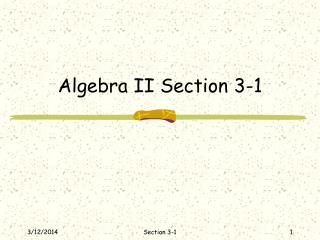DownloadDownload PresentationAlgebra II Section 3-1

# Algebra II Section 3-1

Télécharger la présentation## Algebra II Section 3-1

- - - - - - - - - - - - - - - - - - - - - - - - - - - E N D - - - - - - - - - - - - - - - - - - - - - - - - - - -
##### Presentation Transcript

1. Algebra II Section 3-1 Section 3-1

2. Objectives • Solve systems of linear equations by graphing. • Determine whether a system of linear equations is consistent and independent, consistent and dependent, or inconsistent Section 3-1

3. System of Equations • A system of equations is two or more equations with the same variables. • The solution to a system of equations is an ordered pair; the ordered pair should be in alphabetical order. Section 3-1

4. Solve by Graphing • Solve the system of equations by graphing x – 2y = 0 x + y = 6 Section 3-1

5. Types of Solutions • Consistent if has at least one solution. • Inconsistent if has no solutions. Section 3-1

6. Consistent • Independent if has exactly one solution. • Dependent if has an infinite number of solutions Section 3-1

7. Example #1 • Graph the system of equations and describe it as consistent and independent, consistent and dependent, or inconsistent. x – y = 5 x + 2y = - 4 Section 3-1

8. Example #2 • Graph the system of equations and describe it as consistent and independent, consistent and dependent, or inconsistent. 15x – 6y = 0 5x – 2y = 10 Section 3-1

9. Application A service club is selling copies of their holiday cookbook to raise funds for a project. The printer’s set-up charge is \$200, and each book costs \$2 to print. The cookbooks will sell for \$6 each. How many cookbooks must the members sell before they make a profit? Section 3-1